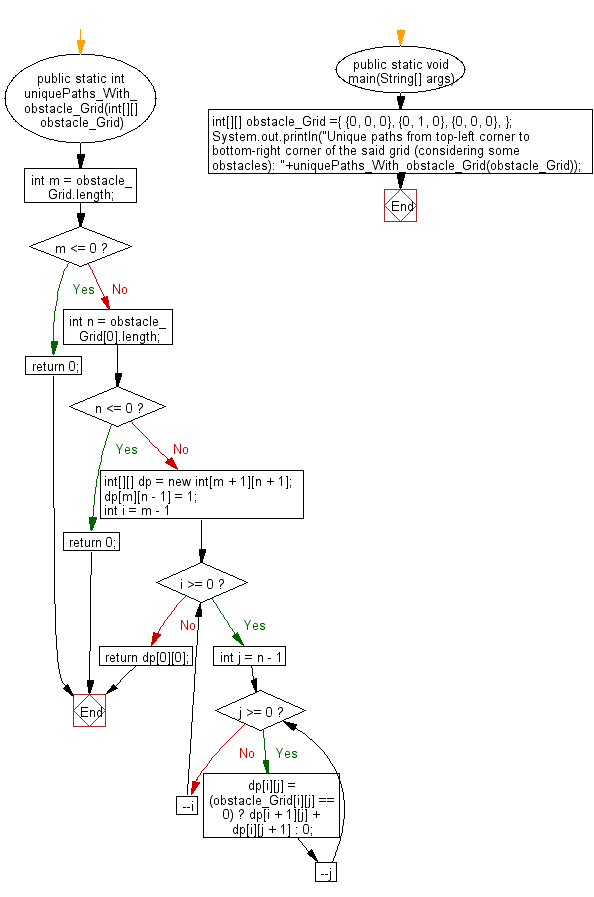﻿ Java: Paths from top-left to bottom-right corner of a grid# Java Exercises: Find possible unique paths considering some obstacles, from top-left corner to bottom-right corner of a specified grid

## Java Basic: Exercise-137 with Solution

Write a Java program to find possible unique paths considering some obstacles, from top-left corner to bottom-right corner of a given grid (m x n).

Note: You can move either down or right at any point in time and an obstacle and empty space is marked as 1 and 0 respectively in the grid. Sample grid:
int[][] obstacle_Grid ={
{0, 0, 0},
{0, 1, 0},
{0, 0, 0},
};

Sample Solution:

Java Code:

``````public class Solution {
public static int uniquePaths_With_obstacle_Grid(int[][] obstacle_Grid) {
int m = obstacle_Grid.length;
if (m <= 0) {
return 0;
}
int n = obstacle_Grid.length;
if (n <= 0) {
return 0;
}
int[][] dp = new int[m + 1][n + 1];
dp[m][n - 1] = 1;
for (int i = m - 1; i >= 0; --i) {
for (int j = n - 1; j >= 0; --j) {
dp[i][j] = (obstacle_Grid[i][j] == 0) ? dp[i + 1][j] + dp[i][j + 1] : 0;
}
}
return dp;
}

public static void main(String[] args) {
int[][] obstacle_Grid ={
{0, 0, 0},
{0, 1, 0},
{0, 0, 0},
};
System.out.println("Unique paths from top-left corner to bottom-right corner of the said grid (considering some obstacles): "+uniquePaths_With_obstacle_Grid(obstacle_Grid));
}
}
```
```

Sample Output:

```Unique paths from top-left corner to bottom-right corner of the said grid (considering some obstacles): 2s
```

Flowchart:Java Code Editor:

Company:

What is the difficulty level of this exercise?

Test your Programming skills with w3resource's quiz.

﻿

## Java: Tips of the Day

countOccurrences

Counts the occurrences of a value in an array.

Use Arrays.stream().filter().count() to count total number of values that equals the specified value.

```public static long countOccurrences(int[] numbers, int value) {
return Arrays.stream(numbers)
.filter(number -> number == value)
.count();
}
```

Ref: https://bit.ly/3kCAgLb